## Selina Concise Mathematics Class 7 ICSE Solutions Chapter 19 Congruency: Congruent Triangles

Selina Publishers Concise Mathematics Class 7 ICSE Solutions Chapter 19 Congruency: Congruent Triangles

APlusTopper.com provides step by step solutions for Selina Concise ICSE Solutions for Class 7 Mathematics. You can download the Selina Concise Mathematics ICSE Solutions for Class 7 with Free PDF download option. Selina Publishers Concise Mathematics for Class 7 ICSE Solutions all questions are solved and explained by expert mathematic teachers as per ICSE board guidelines.

POINTS TO REMEMBER

1. Meaning of Congruency : If two geometrical figures coincide exactly, by placing one over the other, the figures are said to be congruent to each other.
1. Two lines AB and CD are said to be congruent if, on placing AB on CD, or CD on AB ; the two AB and CD exactly coincide.
It is possible only when AB and CD are equal in length.Two figures ABCD and PQRS are said to be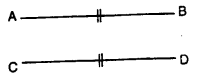congruent if, on placing ABCD on PQRS or PQRS on ABCD the two figures exactly coincide i.e. A and P coincide. B and Q coincide, C and R coincide and D and S coincide.
It is possible only when :
AB = PQ, BC = QR, CD = RS and AD = PS
Also, ∠A = ∠P, ∠B = ∠Q, ∠C = ∠R and ∠D = ∠S.2. Congruency in Triangles : Let triangle ABC is placed over triangle DEF ; such that, vertex A falls on vertex D and side AB falls on side DE ; then if the two triangles coincide with each other in such a way that B falls on E ; C falls on F ; side BC coincides with side EF and side AC coincides with side DF, then the two triangles are congruent to each other.
The symbol used for congruency is “ ≡ ” or “ ≅ ”
∴∆ ABC is congruent to ∆ DEF is written as :
∆ ABC = ∆ DEF or ∆ABC = ∆DEF.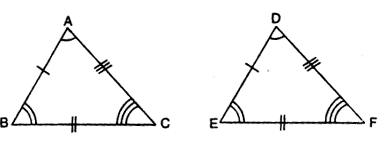3. Corresponding Sides and Corresponding Angles : In case of congruent triangles ABC and DEF, drawn above ; when A ABC is placed over A DEF to cover it exactly; then the sides of the two triangles, which coincide with each other, are called corresponding sides. ‘ Thus, the side AB and DE are corresponding sides; sides BC and EF are corresponding sides and sides AC and DF are also corresponding sides.
In the same way. the angles of the two triangles which coincide with each other, are called corresponding angles. Thus, three pairs of corresponding angles are ∠A and ∠D ; ∠B and ∠E and also ∠C and ∠F.
Note : The corresponding parts of congruent triangles are always equal (congruent).
∴(i) AB = DE, BC = EF and AC = DF. i.e. corresponding sides are equal.
Also (ii) ∠A = ∠D, ZB = ∠E and ∠C = ∠F
i.e. corresponding angles are equal.
4. Conditions of Congruency:
1. If three sides of one triangle are equal to three sides of the other triangle, each to each, then the two triangles are congruent.
The test is known as : side, side, side and is abbreviated as S.S.S.
In triangle ABC and PQR, given alongside:
AB = PQ ; BC = QR and AC = PR
And, so A ABC is congruent to ∆ PQR e. ∆ ABC = ∆ PQR by S.S.S.
Because in congruent triangles, corresponding sides and corresponding angles are equal.
∴ ∠A = ∠P : ∠B = ∠Q and ∠C =∠R2. If two sides and the included angle of one triangle are equal to two sides and the included angle of the other triangle, each to each, then the triangles are congruent.
This test is known as : side, angle, side and is abbreviated as S.A.S. In the given triangles, AB = XZ ; BC = XY and ∠ABC = ∠ZXY
∴ ∆ ABC ≅ ∆ ZXY
Note : Triangles will be congruent by S.A.S., only when the angles included by the corresponding equal sides are equal.
The pairs of corresponding sides of these two congruent triangles are : AB and ZX ; BC and XY ; AC and ZY
The pairs of corresponding angles are :
∠B and ∠X ; ∠A and ∠Z : ∠C and ∠Y.3. If two angles and the included side of one triangle are equal to the two angles and the included side of the other triangle ; then the triangles are congruent.
This test is known as : angle, side, angle and is abbreviated as A.S.A.
In the given figure :
BC = QR;
∠B = ∠Q and ∠C = ∠R
∴ ∆ABC = ∆ PQR.. (by A.S.A.)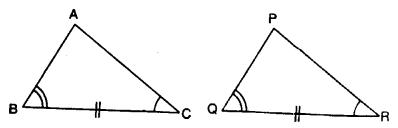4. If the hypotenuse and one side of a right angled triangle are equal to the hypotenuse and one side of another right angled triangle, then the two triangles are congruent.
This test is known as : right angle, hypotenuse, side and is abbreviated as R.H.S.
In the given figure :
∠B = ∠E = 90° ; AB = FE
and hypotenuse AC = hypotenuse FD
∴ ∆ ABC = ∆ FED (byRH.S.)
The corresponding angles in this case are :
∠A and ∠F ; ∠B and ∠E ; ∠C and ∠D and the corresponding sides are :
AB and EF ; AC and FD ; BC and ED.
Since the triangles are congruent, therefore all its corresponding sides are equal and corresponding angles are also equal.
∴ BC = ED ; ∠A = ∠F and ∠C = ∠D
Note : If three angles of a triangle are equal to the three angles of the other triangle, then the triangle are not necessarily congruent.
For congruency at least one pair of corresponding sides must be equal.
∴A.A.A. is not a test of congruency.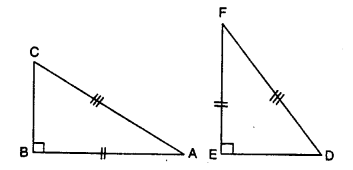### Congruency: Congruent Triangles Exercise 19 – Selina Concise Mathematics Class 7 ICSE Solutions

Question 1.
State, whether the pairs of triangles given in the following figures are congruent or not: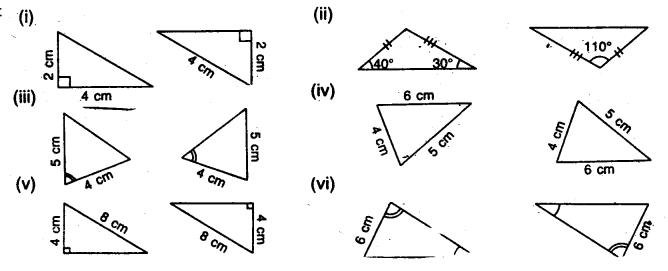Solution:
(i) In these triangles, corresponding sides are not equal. Hence these are not congruent triangles.
(ii) In the first A, third angle
= 180°-(40°+ 30°)
= 180° – 70°
= 110°
Now in these two triangles the sides and included angle of the one are equal to the corresponding sides and included angle.
Hence these are congruent triangles
(S.A.S. axiom)
(iii) In these triangles, corresponding two sides are equal but included angles are not-equal. Hence these are not congruent triangles.
(iv) In these triangles, corresponding three sides are equal.
Hence these are congruent triangles.
(S.S.S. Axiom)
(v) In these right triangles, one side and diagonal of the one, are equal to the corresponding side and diagonal are equal. Hence these are congruent triangles. –
(R.H.S. Axiom)
(vi) In these triangles two sides and one angle of the one are equal to the corresponding sides and one angle of the other are equal.
Hence these are congruent triangles.
(S.S. A. Axiom).
(vii) In A ABC. AB = 2 cm, BC = 3.5 cm and ∠C = 80° and in ∆ DEF,
DE = 2 cm, DF = 3.5 cm and ∠D = 80°From the figure  we see that two corresponding sides  are equal but their included angles are not equal.
Hence, these are not congruent triangles

Question 2.
In the given figure, prove that:
∆ABD ≅ ∆ ACD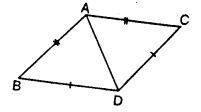Solution: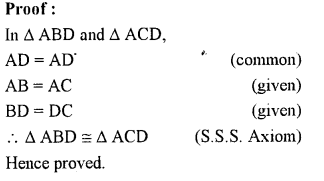Question 3.
Prove that:
(ii) ∠B = ∠D
(iii) AC bisects angle DCB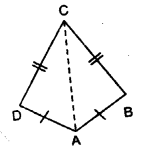Solution: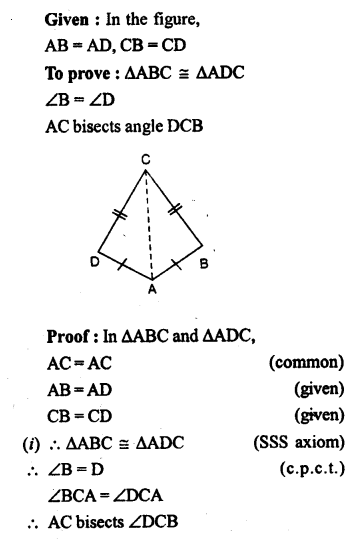Question 4.
Prove that:
(i) ∆ABD  ≡ ∆ACD
(ii) ∠B = ∠CSolution: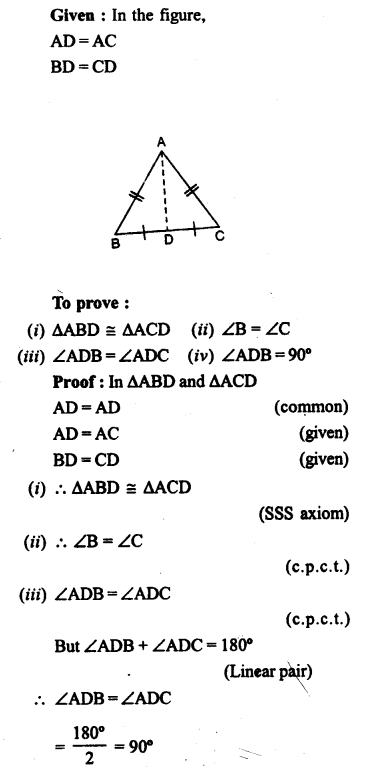Question 5.
In the given figure, prove that:
(i) ∆ACB ≅ ∆ECD
(ii) AB = ED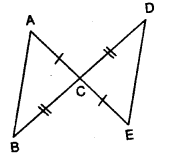Solution: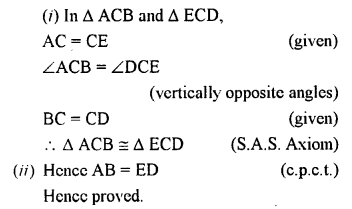Question 6.
Prove that:
(i) ∆ ABC ≅ ∆ ADC
(ii) ∠B = ∠DSolution: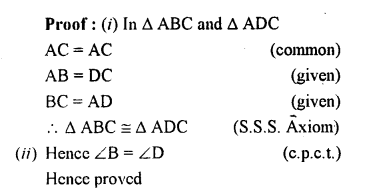Question 7.
In the given figure, prove that: BD = BC.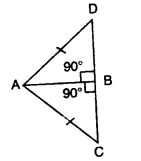Solution:Question 8.
In the given figure ;
∠1 = ∠2 and AB = AC. Prove that:
(i) ∠B = ∠C
(ii) BD = DC
(iii) AD is perpendicular to BC.Solution:Question 9.
In the given figure prove tlyat:
(i) PQ = RS
(ii) PS = QR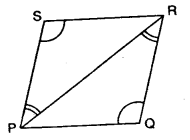Solution:Question 10.
(i) ∆ XYZ ≅ ∆ XPZ
(ii) YZ = PZ
(iii) ∠YXZ = ∠PXZSolution:Question 11.
In the given figure, prove that:
(i) ∆ABC ≅ ∆ DCB
(ii) AC=DB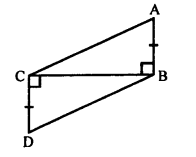Solution: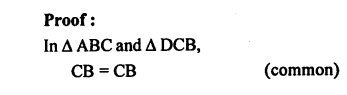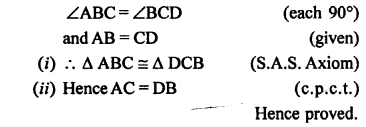Question 12.
In the given figure, prove that:
(i) ∆ AOD ≅ ∆ BOCSolution:Question 13.
ABC is an equilateral triangle, AD and BE are perpendiculars to BC and AC respectively. Prove that:
(ii)BD = CE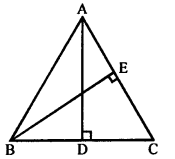Solution:Question 14.
Use the informations given in the following figure to prove triangles ABD and CBD are congruent.
Also, find the values of x and y.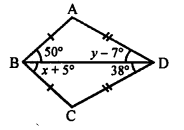Solution: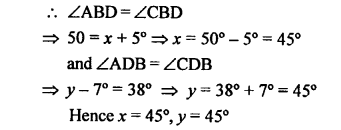Question 15.
The given figure shows a triangle ABC in which AD is perpendicular to side BC and BD = CD. Prove that:
(i) ∆ABD ≅ ∆ACD
(ii) AB=AC
(iii) ∠B = ∠CSolution: